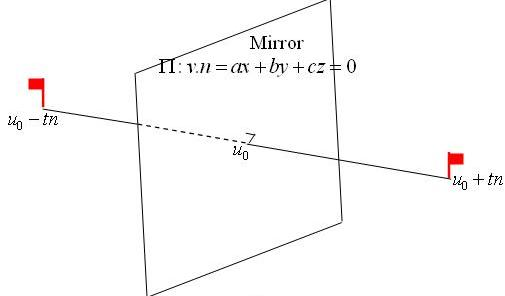#### You may also likeA quadrilateral changes shape with the edge lengths constant. Show the scalar product of the diagonals is constant. If the diagonals are perpendicular in one position are they always perpendicular?As a quadrilateral Q is deformed (keeping the edge lengths constnt) the diagonals and the angle X between them change. Prove that the area of Q is proportional to tanX.### Air Routes

Find the distance of the shortest air route at an altitude of 6000 metres between London and Cape Town given the latitudes and longitudes. A simple application of scalar products of vectors.

# Quaternions and Reflections

##### Age 16 to 18Challenge Level

Quaternions are 4-dimensional numbers of the form $(a,x,y,z)= a+x{\bf i}+y{\bf j}+z{\bf k}$ where $a, x, y$ and $z$ are real numbers, ${\bf i, j}$ and ${\bf k}$ are all different square roots of $-1$ and ${\bf i j} = {\bf k} = {\bf -j i},\ {\bf j k} = {\bf i} = {\bf -k j},\ {\bf k i} = {\bf j} = {\bf -i k}.$

The quaternion $a + x{\bf i} + y{\bf j} + z{\bf k}$ has a real part $a$ and a pure quaternion part $x{\bf i} + y{\bf j}+ z{\bf k}$ where ${\bf i, j}$, and ${\bf k}$ are unit vectors along the axes in ${\bf R^3}$.

(1) For the pure quaternions $v_1 = x_1{\bf i}+y_1{\bf j} + z_1{\bf k}$ and $v_2 = x_2{\bf i} +y_2{\bf j} +z_2{\bf k}$ evaluate the quaternion product $v_1v_2$ and compare your answer to the scalar and vector products $v_1 \cdot v_2$ and $v_1 \times v_2$.

(2) Evaluate the quaternion product $v^2$ where $v=x{\bf i} + y{\bf j} + z{\bf k}$ and $|v| = \sqrt (x^2 + y^2 + z^2) = 1$.

Show that, for all real angles $\theta$ and $\phi$, $$v = \cos \theta \cos \phi {\bf i} + \cos \theta \sin \phi {\bf j} + \sin \theta {\bf k}$$ is a square root of -1. This gives the set of all the points on the unit sphere in ${\bf R^3}$ and shows that the quaternion $-1$ has infinitely many square roots (which we call unit pure quaternions ).(3) Take any unit pure quaternion $n$ ($n^2=-1$) and consider the plane $\Pi$ through the origin in ${\bf R^3}$ with normal vector $n$. Then the plane $\Pi$ has equation $a x + b y + c z = 0 = v\cdot n$. If $u_0$ is a point on the plane $\Pi$ then $u_0\cdot n =0$ and the points $u_0+ t n$ and $u_0 - t n$ are reflections of each other in the plane.

Show that the quaternion map $F(u) = n u n$ gives reflection in the plane $\Pi$ by showing:

(i)$u_0n = -n u_0$ and hence $F(u_0)=u_0$ so that all points on the plane are fixed by this mapping, and

(ii) $F(u_0 + t n) = u_0 - t n$ for all scalars $t$.

If you want to know how quaternions are used in computer graphics and animation in film making read the Plus Article Maths goes to the movies .